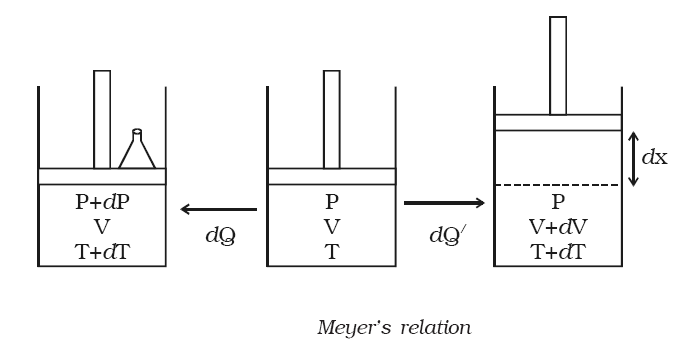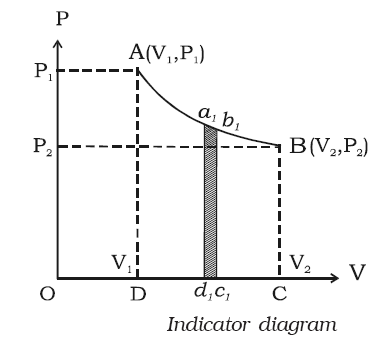×#### Thank you for registering.

One of our academic counsellors will contact you within 1 working day.

Click to Chat

1800-1023-196

+91-120-4616500

CART 0

• 0

MY CART (5)

Use Coupon: CART20 and get 20% off on all online Study Material

ITEM
DETAILS
MRP
DISCOUNT
FINAL PRICE
Total Price: Rs.

There are no items in this cart.
Continue Shopping• Complete Physics Course - Class 11
• OFFERED PRICE: Rs. 2,968
• View Details

Relation between Cp and Cv (Meyer’s relation)

Let us consider one mole of an ideal gas enclosed in a cylinder provided with a frictionless piston of area A. Let P, V and T be the pressure, volume and absolute temperature of gas respectively as shown in below figure.

A quantity of heat dQ is supplied to the gas. To keep the volume of the gas constant, a small weight is placed over the piston. The pressure and the temperature of the gas increase to P + dP and T + dT respectively. This heat energy dQ is used to increase the internal energy dU of the gas. But the gas does not do any work (dW = 0).

∴ dQ = dU = 1 × Cv × dT                …... (1)

The additional weight is now removed from the piston. The piston now moves upwards through a distance dx, such that the pressure of the enclosed gas is equal to the atmospheric pressure P. The temperature of the gas decreases due to the expansion of the gas.Now a quantity of heat dQ’ is supplied to the gas till its temperature becomes T + dT. This heat energy is not only used to increase the internal energy dU of the gas but also to do external work dW in moving the piston upwards.

∴ dQ’  = dU + dW

Since the expansion takes place at constant pressure,

dQ ′ = CpdT

∴ CpdT = CvdT + dW                     …... (2)

Work done, dW = force × distance = = P × A × dx

dW = P dV (since A × dx = dV, change in volume)

∴ CpdT = CvdT + P dV             …... (3)

The equation of state of an ideal gas is

PV = RT

Differentiating both the sides

PdV = RdT              …... (4)

Substituting equation (4) in (3),

CpdT = CvdT + RdT

Cp = Cv + R…... (5)

This equation is known as Meyer’s relation.

Refer this video to know more about on, “Relation between Cp and Cv (Meyer’s relation)”.

Indicator diagram (P-V diagram)A curve showing variation of volume of a substance taken along the X-axis and the variation of pressure taken along Y-axis is called an indicator diagram or P-V diagram. The shape of the indicator diagram shall depend on the nature of the thermodynamical process the system undergoes.

Let us consider one mole of an ideal gas enclosed in a cylinder fitted with a perfectly frictionless piston. Let P1, V1 and T be the initial state of the gas. If dV is an infinitesimally small increase in volume of the gas during which the pressure P is assumed to be constant, then small amount of workdone by the gas is dW = PdV

In the indicator diagram dW = area a1b1c1d1

∴ The total workdone by the gas during expansion from V1 to V2 is

Which is equal to area ABCD, in the indicator diagram.

Hence, in an indicator diagram the area under the curve represents the work done as shown in figure.No external work is being done when a gas is heated at constant volume i.e. gas uses all the haet which is given to it for increasing its internal energy.

If temperature of one mole of agas is raised through 1oC, the molar heat capacity is given itself at constant volume by increase in internal energy.

The Molar Heat capacity of a gas at constant pressure must be greater than Molar Heatcapacity of a gas at constant volume.

The specific heats of gases are given as CP and CV at constant pressure and constant volume respectively while solids and liquids are having only single value for specific heat

When gas is heated through 1oC at constant pressure, the difference between these will give the work done by one mole of the gas in expansion.

The difference between molar heat capacity of a gas at constant pressure, CP and at constant volume, CV is equal to the gas constant R. i.e. 1.987 cal or 8.314 J.

A small electric immersion heater is used to boil 136 g of water for a cup of instant coffee. The heater is labeled 220 watts. Calculate the time required to bring this water from 23.5ºC to the boiling point, ignoring nay heat losses.

Solution:

Heat Q that must be given to a body of mass m, whose material has a specific heat c, to increase its temperature from initial temperature Ti to final temperature Tf is,

Q = mc (Tf - Ti)

But time (t) is equal to the heat energy (Q) divided by power (P).

t = Q/ P

= mc (Tf - Ti)/P

To obtain the time required to bring this water from 23.5̊ C to the boiling point, substitute 136 g for mass of water m, 4190 J/kg. K for specific heat capacity of water c, 100̊ C for final temperature Tf (boiling point of water), 23.5̊ C for initial temperature Ti and 220 watts for power P in the equation t = mc (Tf - Ti)/P,

t = mc (Tf - Ti)/P

= (136 g) (4190 J/kg. K) (100̊ C - 23.5̊ C) / 220 W

= (136 g×10-3 kg/1 g) (4190 J/kg. K) (100̊ C - 23.5̊ C) / 220 W

= (0.136 kg) (4190 J/kg. K) ((100+273) K – (23.5 + 273)K) / 220 W

= (0.136 kg) (4190 J/kg. K) (373 K – 296.5 K) / 220 W

= (0.136 kg) (4190 J/kg. K) (76.5 K) / 220 W

= 198.15 s

Rounding off to three significant figures, the time required to bring this water from 23.5̊ C to the boiling point would be 198 s.Question 1

Gas molecules of different masses in the same container have the same average transnational kinetic energy which is directly proportional to their

(a) volume                                (b) pressure

(c) absolute temperature         (d) time

Question 2

The area enclosed by the curve ABCDA for a Carnot heat engine represents the work done by Carnot engine

(a) at any instant               (b) averagely

(c) during its operation       (d) during one cycle

Question 3

For a gas obeying Boyles law if the pressure is doubled the volume becomes

(a) double          (b) one half

(c) four times     (d) one fourth

Question 4

Which of the following properties of molecules of a gas is same for all gases at particular temperature?

(a) momentum           (b) mass

(c) velocity                 (d) kinetic energy

Question 5

In which process entropy remains constant

(a) isobaric               (b) isochoricQ.1
Q.2
Q.3
Q.4
Q.5

c

d

b

d

c

Related Resources:-

You might like to thermodynamics.

For getting an idea of the type of questions asked, refer the  Previous Year Question Papers.### Course Features

• 101 Video Lectures
• Revision Notes
• Previous Year Papers
• Mind Map
• Study Planner
• NCERT Solutions
• Discussion Forum
• Test paper with Video Solution